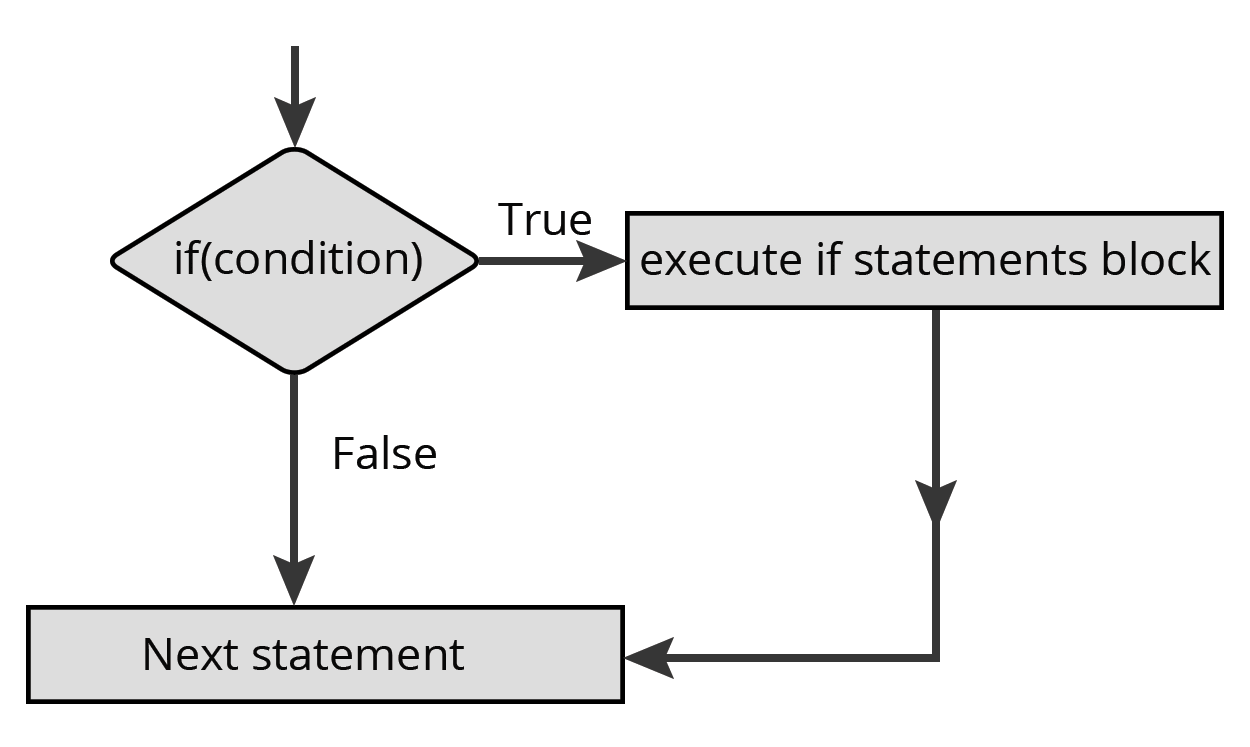# Contents

• Python Tokens
• Variables and Assignments
• Simple Input/Output
• Data Types
• Mutable and Immutable Types
• Expressions
• If conditions
• Loops
• More Loops Lol

# Python Tokens

The smallest unit in a program

## Different Types of Tokens

• keyword
• Identifiers
• Literals
• Operators
• Punctuators

### Keywords

Predefined words which has special meaning to the python interpreter. you can't use this to name your variables

Example Keywords

• False
• True
• None
• import
• pass
• break

there are more, but I am lazy to type it all :)

### Identifiers

The names given by you is an identifier! example? the name of the variables and name of the functions.. there are more.. but I am lazy :)

#### Thou shalt follow the rules

• Variables should be non keyword with no space in between
``````# Invalid Variable
pass variable = "Hello"

# Valid Variable
variable = "World"
``````
• made only with Letters, Numbers and Underscore (_)
``````# Invalid Variable
var-3 = "Hello"

# Valid Variable
var3 = "World"
``````
``````# Invalid Variable
3var = "Hello"

# Valid Variable
var3 = "World"
``````

### Literals

Data items which has fixed/constant value.

#### String Literals

String, just imagine that its a word.

• Single line Strings
``````var = "This is a string"
var1 = 'String with single quotes'
``````
• Multiline Strings
``````var = '''
hello
I am a multi line
string
'''
``````

### Numeric Literals

numeric literals? they are just numbers..

### Integers

`int` they are just integers. 0, positive and negative numbers

Types

• Decimal - Base 10
• Octal - Base 8

### Float

`float` they are numbers with decimal points `3.14159`,`10.32`, etc

### Boolean Literals

its just `True` and `False` nothing much tosay to be honest

### None

`None`. its just none. if you asked something to python and python cannot find it, it returns `None`

## Data Types

### Lists

A group of comma seperated values of any datatypes between square brackets

``````lis = [1,2,3,4,5,6,7,8,9]
lis1 = ['q','w','e']
lis2 = [1,2,3,4,'1']
``````

### Tuples

A group of comma seperated values of any datatypes within parentheses.

``````tup = (1,2,3,4,5)
tup1 = ('q','w','e')
tup2 = (1,2,3,'e')
``````

### Sets

Mutable datatype like `list` but you can't have duplicates in it.

``````# Invalid Set
set1 = {1,1,3,4}

# Valid Set
set2 = {1,2,3,4}
``````

### Dictionaries

an unordered set of comma seperated `key:value` pairs within `{}`

``````dicto = {'a':1 ,'e':2 ,'i':3 ,'o':4}
``````

## If conditions

Plain If condition

``````var = True

if var:
print("Var is True")
``````

You can also write it as.

``````var = True

if var == True:
print("var is True")
``````

## If-else statements``````var = True

if var == True:
print("Var is true")

else:
print("var is false")
``````

## If-elif statements``````var = "Hello"

if var == "Hello":
print("its hello")

elif var == "ok":
print("hi")

else:
print("hmm")
``````